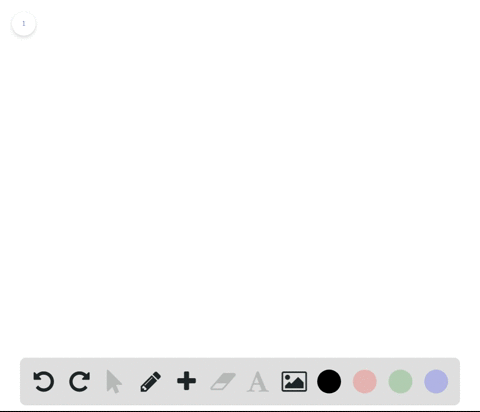Enroll in one of our FREE online STEM summer camps. Space is limited so join now!View Summer Courses### Finding $\delta$ When using the definition of lim…

00:43Need more help? Fill out this quick form to get professional live tutoring.

Get live tutoring
Problem 62

Modeling Data Repeat Exercise 61 for

$C(t)=5.79-0.99[1-t], \quad t>0$

Check back soon!

## Discussion

You must be signed in to discuss.

## Video Transcript

we're giving the following function. C A. T is equal to faculty. Someone on my 0.99 double brackets. A one minus t already double back. Get to represent T T to the greatest whole number. So the grace imager, um, where t is greater than zero. So to sign off, let's for part A were asked determine what sea of 10 points on five is equal to. So you have a sea of 10.75 is equal to 5.79 minus 0.99 of one minus ton points. Other thoughts All right? And so we can simplify. Gerston is equal to 5.79 Well, this sterile 0.99 of negative nine point Southern. Well, you know that this is leaves to the nearest whole number. So we end up with 10. So we have 5.79 minus 0.99 to 90 ton, which gets us 5.75 We're sorry. Not factually. So 5.7 nine. 5.79 plus 9.99 which is equal to 15.6 sign. So we know that after 10.75 minutes, it costs \$15.69. All right, so far. Second part, we are to determine if the language for perky we're to the term reign. If the limit as t approaches history of C A T exist so we can look at a graph. So this is a function we can see that that's about three right over here, right here. Between these two, delimit does not exist because there's a gap, so we know that the warm it does not exist.